‎14754‎ مشاهدة
Updated Indicator - Plots High, Low Open, Close

For Weekly, Daily, 4 Hour, 2 Hour, 1 Hour Current and Previous Sessions Levels.

Updated Adds 4 Hour, 2 Hour, 1 Hour levels for Forex and Intra-Day Traders.
//Created By ChrisMoody on 6-14-2014 -- Updated 1-29-2015
//Updated for Forex and Intraday traders.  No plots Current and Previous Sessions Data based on Weekly, Daily, 4 hour, 2 hour, 1 hour OHLC
//Daily Session To be used on IntraDay Charts
swt = input(false, title="Show This Weeks OHLC?")
swy = input(false, title="Show Previous Weeks OHLC?")
st = input(true, title="Show Todays OHLC?")
sy = input(false, title="Show Yesterdays OHLC?")
stf = input(false, title="Show 4-Hour OHLC?")
syf = input(false, title="Show Previous 4-hour OHLC?")
stt = input(false, title="Show 2-Hour OHLC?")
syt = input(false, title="Show Previous 2-hour OHLC?")
sto = input(false, title="Show 1-Hour OHLC?")
syo = input(false, title="Show Previous 1-hour OHLC?")

//Weekly
wtdo = security(tickerid, 'W', open)
wpdo = security(tickerid, 'W', open)
wpc = security(tickerid, 'W', close)
wpdc = security(tickerid, 'W', close)
wph = security(tickerid, 'W', high)
wpdh = security(tickerid, 'W', high)
wpl = security(tickerid, 'W', low)
wpdl = security(tickerid, 'W', low)
//Daily
tdo = security(tickerid, 'D', open)
pdo = security(tickerid, 'D', open)
pc = security(tickerid, 'D', close)
pdc = security(tickerid, 'D', close)
ph = security(tickerid, 'D', high)
pdh = security(tickerid, 'D', high)
pl = security(tickerid, 'D', low)
pdl = security(tickerid, 'D', low)
//4 Hour
ftdo = security(tickerid, '240', open)
fpdo = security(tickerid, '240', open)
fpc = security(tickerid, '240', close)
fpdc = security(tickerid, '240', close)
fph = security(tickerid, '240', high)
fpdh = security(tickerid, '240', high)
fpl = security(tickerid, '240', low)
fpdl = security(tickerid, '240', low)
//2 Hour
ttdo = security(tickerid, '120', open)
tpdo = security(tickerid, '120', open)
tpc = security(tickerid, '120', close)
tpdc = security(tickerid, '120', close)
tph = security(tickerid, '120', high)
tpdh = security(tickerid, '120', high)
tpl = security(tickerid, '120', low)
tpdl = security(tickerid, '120', low)
//1 Hour
otdo = security(tickerid, '60', open)
opdo = security(tickerid, '60', open)
opc = security(tickerid, '60', close)
opdc = security(tickerid, '60', close)
oph = security(tickerid, '60', high)
opdh = security(tickerid, '60', high)
opl = security(tickerid, '60', low)
opdl = security(tickerid, '60', low)

//Weekly Plots
plot(swt and wtdo ? wtdo : na, title="Weekly Open", style=circles, linewidth=3, color=silver)
plot(swy and wpdo ? wpdo : na, title="Previous Weeks Open", style=cross, linewidth=3, color=silver)
plot(swt and wpc ? wpc : na, title="Weekly Close", style=circles, linewidth=3, color=fuchsia)
plot(swy and wpdc ? wpdc : na, title="Previous Weeks Close", style=cross, linewidth=3, color=fuchsia)
plot(swt and wph ? wph : na, title="Weekly High", style=circles, linewidth=3, color=green)
plot(swy and wpdh ? wpdh : na, title="Previous Weeks High", style=cross, linewidth=3, color=green)
plot(swt and wpl ? wpl : na, title="Weekly Low", style=circles, linewidth=3, color=red)
plot(swy and wpdl ? wpdl : na, title="Previous Weeks Low", style=cross, linewidth=3, color=red)
//Daily Plots
offs_daily = 0
plot(st and tdo ? tdo : na, title="Daily Open", style=circles, linewidth=2, color=silver)
plot(sy and pdo ? pdo : na, title="Previous Days Open", style=cross, linewidth=2, color=silver)
plot(st and pc ? pc : na, title="Daily Close", style=circles, linewidth=2, color=fuchsia)
plot(sy and pdc ? pdc : na, title="Previous Days Close", style=cross, linewidth=2, color=fuchsia)
plot(st and ph ? ph : na, title="Daily High", style=circles, linewidth=2, color=lime)
plot(sy and pdh ? pdh : na, title="Previous Daily High", style=cross, linewidth=2, color=lime)
plot(st and pl ? pl : na, title="Daily Low", style=circles, linewidth=2, color=red)
plot(sy and pdl ? pdl : na, title="Previous Daily Low", style=cross, linewidth=2, color=red)
//4-Hour Plots
plot(stf and ftdo ? ftdo : na, title="4-Hour Open", style=circles, linewidth=2, color=silver)
plot(syf and fpdo ? fpdo : na, title="Previous 4-Hour Open", style=cross, linewidth=2, color=silver)
plot(stf and fpc ? fpc : na, title="4-Hour Close", style=circles, linewidth=2, color=fuchsia)
plot(syf and fpdc ? fpdc : na, title="Previous 4-Hour Close", style=cross, linewidth=2, color=fuchsia)
plot(stf and fph ? fph : na, title="4-Hour High", style=circles, linewidth=2, color=lime)
plot(syf and fpdh ? fpdh : na, title="Previous 4-Hour High", style=cross, linewidth=2, color=lime)
plot(stf and fpl ? fpl : na, title="4-Hour Low", style=circles, linewidth=2, color=red)
plot(syf and fpdl ? fpdl : na, title="Previous 4-Hour Low", style=cross, linewidth=2, color=red)
//2-Hour Plots
plot(stt and ttdo ? ttdo : na, title="4-Hour Open", style=circles, linewidth=2, color=silver)
plot(syt and tpdo ? tpdo : na, title="Previous 4-Hour Open", style=cross, linewidth=2, color=silver)
plot(stt and tpc ? tpc : na, title="4-Hour Close", style=circles, linewidth=2, color=fuchsia)
plot(syt and tpdc ? tpdc : na, title="Previous 4-Hour Close", style=cross, linewidth=2, color=fuchsia)
plot(stt and tph ? tph : na, title="4-Hour High", style=circles, linewidth=2, color=lime)
plot(syt and tpdh ? tpdh : na, title="Previous 4-Hour High", style=cross, linewidth=2, color=lime)
plot(stt and tpl ? tpl : na, title="4-Hour Low", style=circles, linewidth=2, color=red)
plot(syt and tpdl ? tpdl : na, title="Previous 4-Hour Low", style=cross, linewidth=2, color=red)
//1-Hour Plots
plot(sto and otdo ? otdo : na, title="4-Hour Open", style=circles, linewidth=2, color=silver)
plot(syo and opdo ? opdo : na, title="Previous 4-Hour Open", style=cross, linewidth=2, color=silver)
plot(sto and opc ? opc : na, title="4-Hour Close", style=circles, linewidth=2, color=fuchsia)
plot(syo and opdc ? opdc : na, title="Previous 4-Hour Close", style=cross, linewidth=2, color=fuchsia)
plot(sto and oph ? oph : na, title="4-Hour High", style=circles, linewidth=2, color=lime)
plot(syo and opdh ? opdh : na, title="Previous 4-Hour High", style=cross, linewidth=2, color=lime)
plot(sto and opl ? opl : na, title="4-Hour Low", style=circles, linewidth=2, color=red)
plot(syo and opdl ? opdl : na, title="Previous 4-Hour Low", style=cross, linewidth=2, color=red)

## التعليقات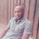Hi Chris, I use FXCM trading station for my charting and I will love to use ur script on there, but I'm not a coder. Any idea on how I can accomplish this? Thanks, great work.
رد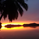Hi Chris, Love your work. Are you able to create a simple Fibonacci indicator based on my preference of where to plot the indicator?

cheers mate
رد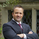Thanks Chris! this will same me personally so much time... 10/10 once again!
رد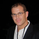IvanLabrie
Beautiful my be a little overstatement....But I'll take it
ردChrisMoody
Oh well, it can be really useful for intraday trading, particularly using stochastics.
Haven't used those for ages though, but still good.
رد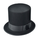Hi Chris. I have been using this indicator for a while and find it helpful. I use it to plot the previous month high and low. Recently I noticed that it seems to be displaying a number of lines on the daily chart that look to be previous weekly highs and lows even though those boxes are unchecked. Any ideas? Thanks
رد